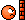# Why is there a time unit in some inertia units and not in others?

Why are some inertia units lb-in-s^2 and others lb-in^2. what the difference? The first one is from a gear box spec and the second is from a motor rotor.

jbriggs444
Homework Helper
Why are some inertia units lb-in-s^2 and others lb-in^2. what the difference? The first one is from a gear box spec and the second is from a motor rotor.
Guessing here...

If you want to use the pound mass for moment of inertia then an appropriate unit could be pound(mass) inch2.

If you want to use the pound force for moment of inertia then you have to first convert it to a unit of mass. For instance, the mass which would be accelerated at a rate of one inch per second squared by a force of one pound force. That unit of mass is also known as a "slinch". (A slug is what you get when you use feet instead of inches. A slinch is what you get when you use inches).

One pound force is one slinch-inch-second2. If you express moment of inertia in slinch-inch2 then that is the same as one pound(force)-inch-second2

[Yeah, yeah, we all know that the U.S. customary system of units is pathetic. No need to crow over it]

jack action
Gold Member
To repeat what @jbriggs444 said with other words (I mean, for us who don't bother with the U.S. customary system):

You could rewrite the presented units this way:
• lbf.in.s²
• lbm.in²
Where one is a pound-force and the other is a pound-mass.

If you do a dimension analysis, the unit for inertia should be M.L² (Mass X Length²).

But from F= ma (or m = F/a), we know that a mass could be defined as F.T²/L (Force X Time² / Length).

Replacing in the inertia unit, we get M.L² = (F.T²/L). L² = F.L.T²; So both units are equivalent.

jbriggs444
Homework Helper
To repeat what @jbriggs444
Replacing in the inertia unit, we get M.L² = (F.T²/L). L² = F.L.T²; So both units are equivalent.
I agree that both units have the proper dimensionality to measure a moment of inertia. But I do not agree that the two units are the same. They are out by a factor of one g expressed in inches per second squared.

Visit, for instance,http://www.translatorscafe.com/cafe/EN/units-converter/moment-of-inertia/12-1/

jack action
Gold Member
They are out by a factor of one g expressed in inches per second squared.
I meant equivalent in the sense that they represent the same dimension (like bar and Pascal for units of pressure for example), but not necessarily by the same factor. Sorry for the confusion.

•jbriggs444
Ok this is a little more clear but if I may follow up with this. I went to this site mentioned above:
http://www.translatorscafe.com/cafe/EN/units-converter/moment-of-inertia/12-1/
and 1 pound-force inch second² = 386.0885865302 pound inch². So if I have a control system with several components I just add the inertia's together but they have to be the same units so all the pound-force inch second² components need to be multiplied by 386.09 in order to get a system inertia. This is all I need to do to get the system inertia, correct? Thanks in advance for your help.

jack action